# Statistics.ppt

31 de May de 2023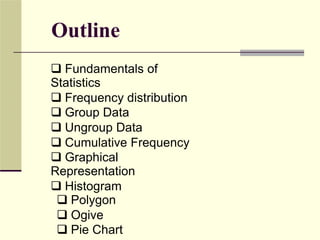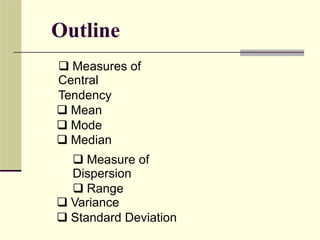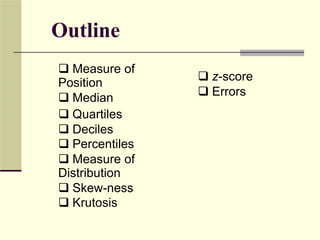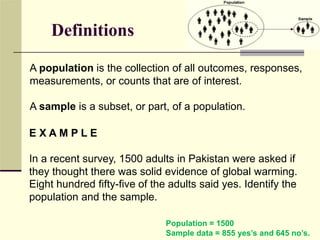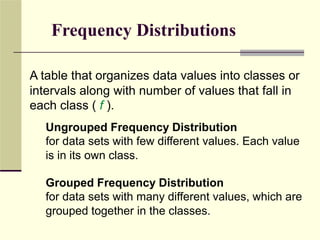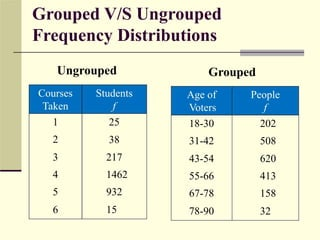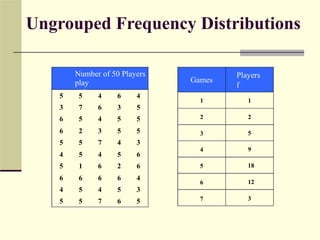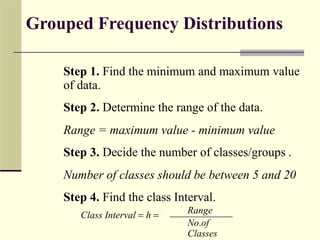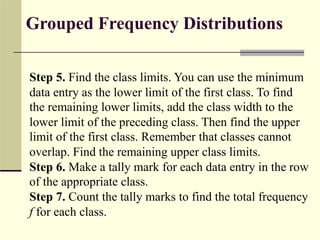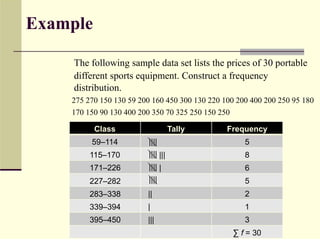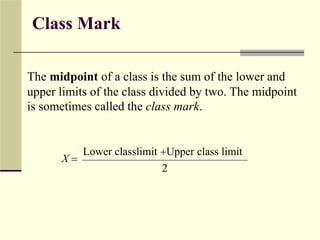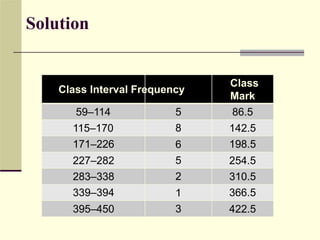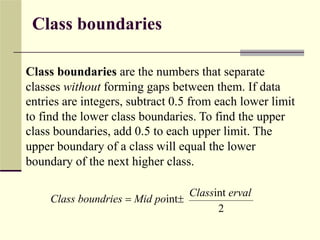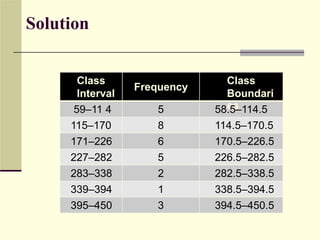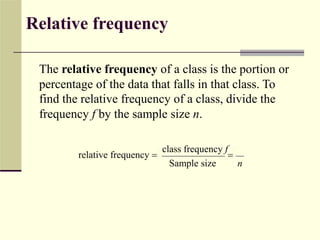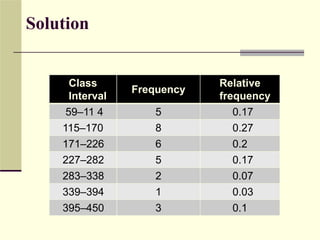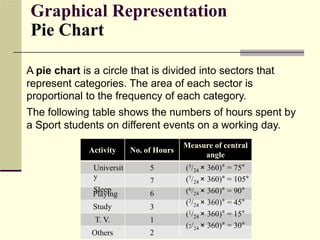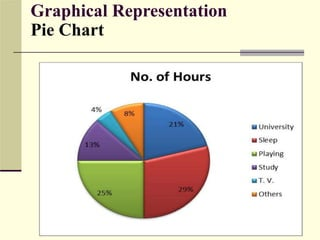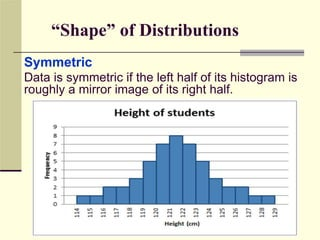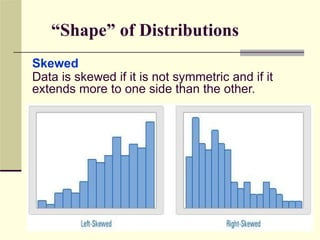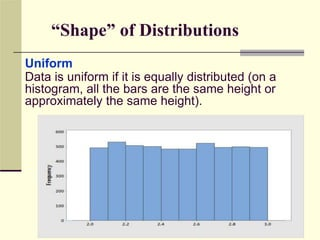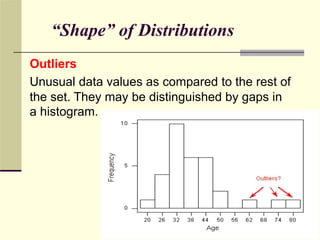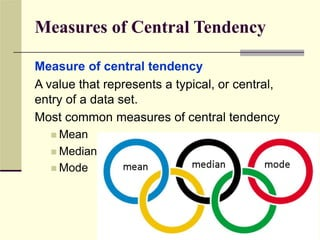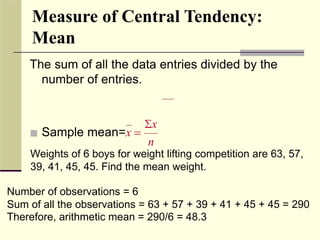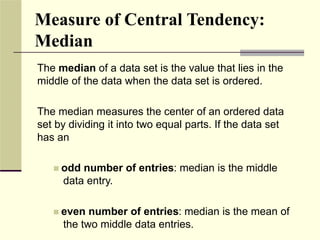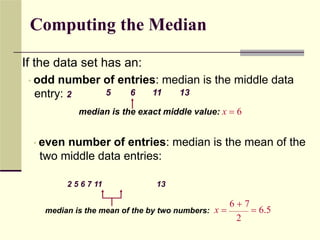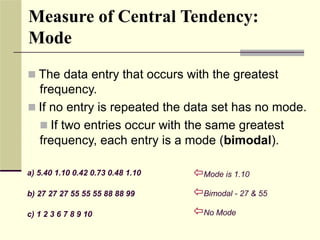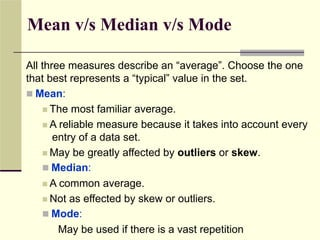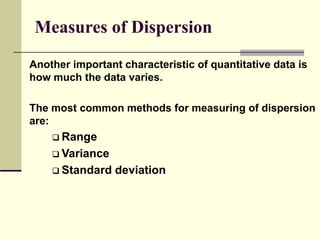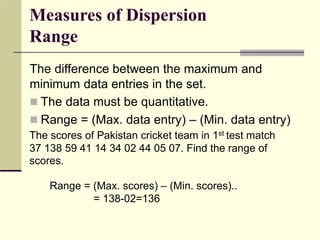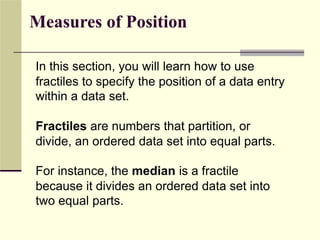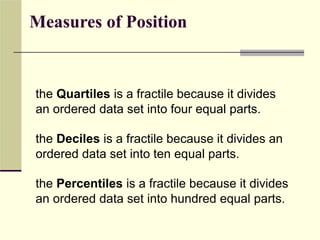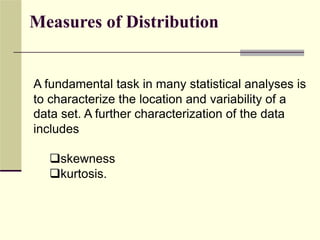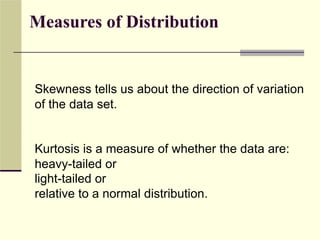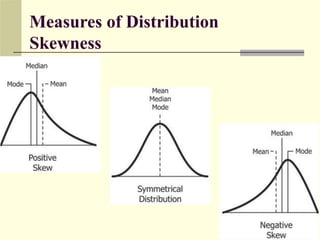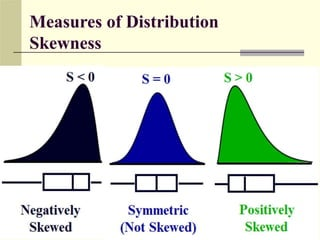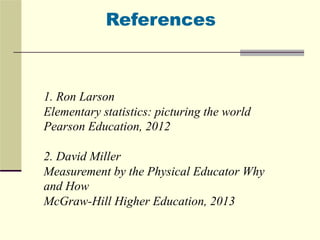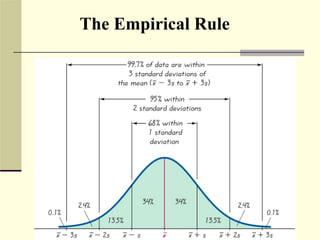1 de 40

### Statistics.ppt

• 2. Outline  Fundamentals of Statistics  Frequency distribution  Group Data  Ungroup Data  Cumulative Frequency  Graphical Representation  Histogram  Polygon  Ogive  Pie Chart
• 3. Outline  Measures of Central Tendency  Mean  Mode  Median  Measure of Dispersion  Range  Variance  Standard Deviation
• 4. Outline  Measure of Position  Median  Quartiles  Deciles  Percentiles  Measure of Distribution  Skew-ness  Krutosis  z-score  Errors
• 5. Definitions A population is the collection of all outcomes, responses, measurements, or counts that are of interest. A sample is a subset, or part, of a population. E X A M P L E In a recent survey, 1500 adults in Pakistan were asked if they thought there was solid evidence of global warming. Eight hundred fifty-five of the adults said yes. Identify the population and the sample. Population = 1500 Sample data = 855 yes’s and 645 no’s.
• 6. Frequency Distributions A table that organizes data values into classes or intervals along with number of values that fall in each class ( f ). Ungrouped Frequency Distribution for data sets with few different values. Each value is in its own class. Grouped Frequency Distribution for data sets with many different values, which are grouped together in the classes.
• 7. Grouped V/S Ungrouped Frequency Distributions Ungrouped Grouped Courses Students Age of People Taken f Voters 18-30 31-42 43-54 55-66 67-78 78-90 f 1 2 3 4 5 6 25 202 508 620 413 158 32 38 217 1462 932 15
• 8. Ungrouped Frequency Distributions Number of 50 Players play different games Players f Games 5 3 6 6 5 4 5 6 4 5 5 7 5 2 5 5 1 6 5 5 4 6 4 3 7 4 6 6 4 7 6 3 5 5 4 5 2 6 5 6 4 5 5 5 3 6 6 4 3 5 1 2 3 4 5 6 7 1 2 5 9 18 12 3
• 9. Grouped Frequency Distributions Step 1. Find the minimum and maximum value of data. Step 2. Determine the range of the data. Range = maximum value - minimum value Step 3. Decide the number of classes/groups . Number of classes should be between 5 and 20 Step 4. Find the class Interval. Range No.of Classes Class Interval  h 
• 10. Grouped Frequency Distributions Step 5. Find the class limits. You can use the minimum data entry as the lower limit of the first class. To find the remaining lower limits, add the class width to the lower limit of the preceding class. Then find the upper limit of the first class. Remember that classes cannot overlap. Find the remaining upper class limits. Step 6. Make a tally mark for each data entry in the row of the appropriate class. Step 7. Count the tally marks to find the total frequency f for each class.
• 11. Example The following sample data set lists the prices of 30 portable different sports equipment. Construct a frequency distribution. 275 270 150 130 59 200 160 450 300 130 220 100 200 400 200 250 95 180 170 150 90 130 400 200 350 70 325 250 150 250 Class Tally Frequency 59–114 |||| |||| |||| |||| 5 115–170 171–226 227–282 283–338 339–394 395–450 ||| | 8 6 5 || | 2 1 3 ||| ∑ f = 30
• 12. Class Mark The midpoint of a class is the sum of the lower and upper limits of the class divided by two. The midpoint is sometimes called the class mark. Lower classlimit Upper class limit X  2
• 13. Solution Class Mark Class Interval Frequency 59–114 5 8 6 5 2 1 3 86.5 115–170 171–226 227–282 283–338 339–394 395–450 142.5 198.5 254.5 310.5 366.5 422.5
• 14. Class boundaries Class boundaries are the numbers that separate classes without forming gaps between them. If data entries are integers, subtract 0.5 from each lower limit to find the lower class boundaries. To find the upper class boundaries, add 0.5 to each upper limit. The upper boundary of a class will equal the lower boundary of the next higher class. Classint erval Class boundries  Mid point 2
• 16. Relative frequency The relative frequency of a class is the portion or percentage of the data that falls in that class. To find the relative frequency of a class, divide the frequency f by the sample size n. class frequency f relative frequency   Sample size n
• 18. Graphical Representation Pie Chart A pie chart is a circle that is divided into sectors that represent categories. The area of each sector is proportional to the frequency of each category. The following table shows the numbers of hours spent by a Sport students on different events on a working day. Measure of central Activity No. of Hours angle Universit y Sleep 5 7 6 3 1 2 (5/24 × 360)° = 75° (7/24 × 360)° = 105° (6/24 × 360)° = 90° (3/24 × 360)° = 45° (1/24 × 360)° = 15° (2/24 × 360)° = 30° Playing Study T. V. Others
• 20. “Shape” of Distributions Symmetric Data is symmetric if the left half of its histogram is roughly a mirror image of its right half.
• 21. “Shape” of Distributions Skewed Data is skewed if it is not symmetric and if it extends more to one side than the other.
• 22. “Shape” of Distributions Uniform Data is uniform if it is equally distributed (on a histogram, all the bars are the same height or approximately the same height).
• 23. “Shape” of Distributions Outliers Unusual data values as compared to the rest of the set. They may be distinguished by gaps in a histogram.
• 24. Measures of Central Tendency Measure of central tendency A value that represents a typical, or central, entry of a data set. Most common measures of central tendency  Mean  Median  Mode
• 25. Measure of Central Tendency: Mean The sum of all the data entries divided by the number of entries. x  Sample mean=x  n Weights of 6 boys for weight lifting competition are 63, 57, 39, 41, 45, 45. Find the mean weight. Number of observations = 6 Sum of all the observations = 63 + 57 + 39 + 41 + 45 + 45 = 290 Therefore, arithmetic mean = 290/6 = 48.3
• 26. Measure of Central Tendency: Median The median of a data set is the value that lies in the middle of the data when the data set is ordered. The median measures the center of an ordered data set by dividing it into two equal parts. If the data set has an  odd number of entries: median is the middle data entry.  even number of entries: median is the mean of the two middle data entries.
• 27. Computing the Median If the data set has an: • odd number of entries: median is the middle data entry: 2 5 6 11 13 median is the exact middle value: x  6 • even number of entries: median is the mean of the two middle data entries: 2 5 6 7 11 13 6  7 x   6.5 median is the mean of the by two numbers: 2
• 28. Measure of Central Tendency: Mode  The data entry that occurs with the greatest frequency.  If no entry is repeated the data set has no mode.  If two entries occur with the same greatest frequency, each entry is a mode (bimodal). Mode is 1.10 a) 5.40 1.10 0.42 0.73 0.48 1.10 b) 27 27 27 55 55 55 88 88 99 c) 1 2 3 6 7 8 9 10 Bimodal - 27 & 55 No Mode
• 29. Mean v/s Median v/s Mode All three measures describe an “average”. Choose the one that best represents a “typical” value in the set.  Mean:  The most familiar average.  A reliable measure because it takes into account every entry of a data set.  May be greatly affected by outliers or skew.  Median:  A common average.  Not as effected by skew or outliers.  Mode: May be used if there is a vast repetition
• 30. Measures of Dispersion Another important characteristic of quantitative data is how much the data varies. The most common methods for measuring of dispersion are:  Range  Variance  Standard deviation
• 31. Measures of Dispersion Range The difference between the maximum and minimum data entries in the set.  The data must be quantitative.  Range = (Max. data entry) – (Min. data entry) The scores of Pakistan cricket team in 1st test match 37 138 59 41 14 34 02 44 05 07. Find the range of scores. Range = (Max. scores) – (Min. scores).. = 138-02=136
• 32. Measures of Position In this section, you will learn how to use fractiles to specify the position of a data entry within a data set. Fractiles are numbers that partition, or divide, an ordered data set into equal parts. For instance, the median is a fractile because it divides an ordered data set into two equal parts.
• 33. Measures of Position the Quartiles is a fractile because it divides an ordered data set into four equal parts. the Deciles is a fractile because it divides an ordered data set into ten equal parts. the Percentiles is a fractile because it divides an ordered data set into hundred equal parts.
• 34. Measures of Distribution A fundamental task in many statistical analyses is to characterize the location and variability of a data set. A further characterization of the data includes skewness kurtosis.
• 35. Measures of Distribution Skewness tells us about the direction of variation of the data set. Kurtosis is a measure of whether the data are: heavy-tailed or light-tailed or relative to a normal distribution.
• 38. References 1. Ron Larson Elementary statistics: picturing the world Pearson Education, 2012 2. David Miller Measurement by the Physical Educator Why and How McGraw-Hill Higher Education, 2013